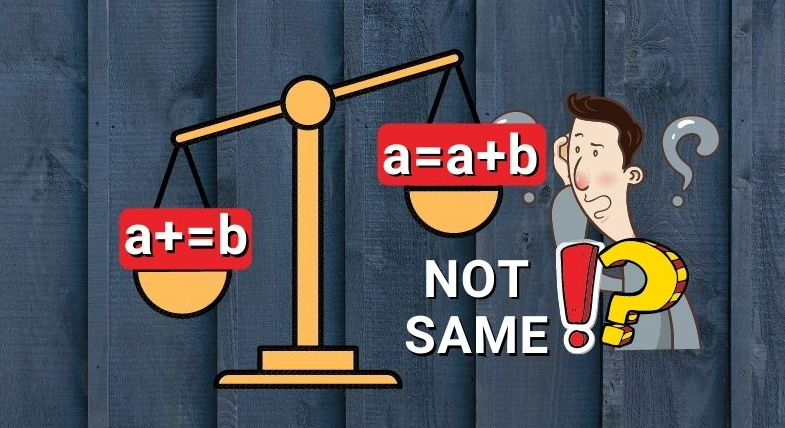Join thousands of AI enthusiasts and experts at the Learn AI Community.

## PublicationProgramming

# The difference between a=a+b and a+=b in Python

Last Updated on November 3, 2021 by Editorial Team

### Introduction

I am sure you are already aware of the concatenation operators + and += in Python. As the name itself says, concatenation operators are used to concatenating multiple sequence types (For example, concatenate two lists). Similarly, * and *= are used when you want to repeat a sequence type n times. You also know that both these produce the same results.

But many Python developers think my_list1 += my_list2 as a shorthand notation to my_list1 = my_list1 + my_list2. The same argument holds true for my_list1 *= n and my_list1 = my_list * n. But its far from true.

In this short article, you are going to learn the difference between + vs += and * vs *=.

### + and +=

Let’s look at an example below using a list. When you try to concatenate two lists mylist_1 and mylist_2 using the + operator, it creates a new object and assigns mylist_1 to the new object.

As you can see mylist_1 and mylist_2 are pointing to two different memory locations. And the result of the concatenation is also pointing to a different memory location.

```>>> mylist_1 = [1, 2, 3]
>>> mylist_2 = [4, 5]
>>> print(id(mylist_1), id(mylist_2))
1614327515784 1614319969800```
```>>> mylist_1 = mylist_1 + mylist_2
>>> print(mylist_1)
>>> print(id(mylist_1))
[1, 2, 3, 4, 5]
1614319969416```

Now, look at the same example but using in-place concatenation operator +=. As you can see, the += operator didn’t create a new object. The mylist_1 is pointing to the same memory location before and after the concatenation. We can say that mylist_1 is mutated.

```>>> mylist_1 = [1, 2, 3]
>>> mylist_2 = [4, 5]
>>> print(id(mylist_1), id(mylist_2))
1614325213896 1614316889416```
```>>> mylist_1 += mylist_2
>>> print(mylist_1)
>>> print(id(mylist_1))
[1, 2, 3, 4, 5]
1614325213896```

However, for immutable objects such as int, floats, string, etc. it doesn’t matter. As seen from the below example, the memory location of num1 is different before and after the concatenation in both scenarios.

```>>> num1 = 10
>>> num2 = 20
>>> id(num1), id(num2)
(140726841418416, 140726841418736)```
```>>> num1 = num1 + num2
>>> id(num1)
140726841419056```
```>>> num1 += num2
>>> id(num1)
140726841419696```

The difference between both the concatenation operators is that the + creates a new list and the += modifies an existing list in place.

### * and *=

The repetition operators also behave similarly to the concatenation operators we just went through. Refer to the example below. The memory location of mylist1 is different before and after the repetition (*) operator.

```>>> mylist1 = [1,2]
>>> id(mylist1)
2020051867784```
```>>> mylist1 = mylist1 * 3
>>> print(mylist1)
[1, 2, 1, 2, 1, 2]
>>> id(mylist1)
2020051883912```

Now, let’s look at the same example using the *= operator. As you can see, the memory location of mylist1 is the same before and after the concatenation operation. So, mylist1 is mutated with the *= operator.

```>>> mylist1 = [1,2]
>>> id(mylist1)
2020051837832```
```>>> mylist1 *= 3
>>> print(mylist1)
[1, 2, 1, 2, 1, 2]
>>> id(mylist1)
2020051837832```

The in-place repetition operator also doesn’t make difference for an immutable object because we can’t modify an immutable object.

The difference between both the repetition operators is that the * creates a new list and the *= modifies an existing list in place.

The main benefit of using in-place concatenation (+=) or in-place repetition (*=) is that it is faster than normal concatenation or repetition operation. Because the in-place operation mutates the object and Python doesn’t have to create a new object which saves some time.

#### Concatenation speed comparison

As seen from the below example, += is marginally faster than the + operator. The + operator took 2.42 seconds whereas the += operator took 2.29 seconds.

+ operator

```>>> code = """
>>> mylist_1 = [1, 2, 3, 4, 5, 7, 8]
>>> mylist_2 = [9, 10]
>>> mylist_1 = mylist_1 + mylist_2
>>> """
>>> import timeit
>>> timeit.timeit(stmt=code, number=10_000_000)
2.428993300000002```

+= operator

```>>> code = """
>>> mylist_1 = [1, 2, 3, 4, 5, 7, 8]
>>> mylist_2 = [9, 10]
>>> mylist_1 += mylist_2
>>> """
>>> import timeit
>>> timeit.timeit(stmt=code, number=10_000_000)
2.293214599999999```

#### Repetition speed comparison

As seen from the below example, *= is marginally faster than the *operator. The * operator took

*operator

```>>> code = """
>>> mylist_1 = [1, 2, 3, 4, 5, 6, 7, 8, 9, 10]
>>> mylist_1 = mylist_1 * 2
>>> """```
```>>> import timeit
>>> timeit.timeit(stmt=code, number=10_000_000)
4.3188133```

*= operator

```>>> code = """
>>> mylist_1 = [1, 2, 3, 4, 5, 6, 7, 8, 9, 10]
>>> mylist_1 *= 2
>>> """```
```>>> import timeit
>>> timeit.timeit(stmt=code, number=10_000_000)
4.291261399999999```

### Conclusion

In this article, you have understood an important difference between `+ and +=` and `* and *=` operators. Both * and + operator create a new list whereas t `+= and *=` modifies an existing list in place.

Originally published at pythonsimplified on June 22, 2021.

Published via Towards AI